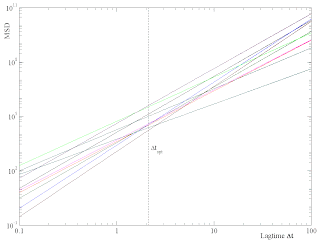## Friday, March 12, 2010

### On the apparent correlations between diffusivity D and power-law exponent b

When analyzing the trajectories of cytoskeleton-bound beads or whole cells, one frequently finds MSD curves as a function of lag time that can be fitted to a power-law within a broad temporal range.

To demonstrate such a power-law regime, one plots the MSD double-logarithmically. To show that analytically, one defines an arbitrary unit of time t0 (for example, t0 = 1 min) and length r0 (for example, r0 = 1 um), in order to make the lagtime and the displacement dimensionless. Then, the MSD curves can be locally written as

\left( \Delta R / r_0 \right)^2 = D \cdot \left( \Delta t / t_0 \right) ^b

The two dimensionless parameters D and b are called the (apparent) "diffusivity" and the "power-law exponent", respectively. The logarithm of the above equation reads

\log\left( ( \Delta R / r_0 )^2 \right) = \log(D) + b \cdot \log( \Delta t / t_0)

Defining

y = \log\left( ( \Delta R / r_0 )^2\right)

and

a =  \log(D)

and

x =  \log( \Delta t / t_0)

we obtain a linear relation for our logarithmic variables:

y = a + b x.

Typical experiments yield a whole bunch of MSD-curves, corresponding to a set of N parameter pairs {(a_i,b_i)}. The a_i and b_i are fluctuating, with the a_i often being normally distributed. When the value-pairs (a_i,b_i) are plotted as a point cloud in the a-b plane, one frequently finds correlations, such as high a-values (=logarithmic diffusivities) coming together with small b-values (=power-law exponents).

However, note that these statistical properties depend on the choice of the length and time units that have been chosen to make the variables dimensionless. In the logarithmic domain, the a-value is just the y-intercept of the linear curve, a=y(x=0). In the original domain, this implies

D = e^a = e^{y(x=0)} =\Delta R^2(\Delta t=t_0) / r_0^2.

Therefore, the distribution P(D) will depend on the parameters t_0 and r_0. For an extreme example, imagine a double-log plot with a bunch of straight MSD lines that all intersect at some point. If we evaluate the distribution P(D) precisely at the lagtime of the crossing point, we will obtain a delta-distribution ! Of course, in reality the lines will not all cross at one point, yet they might approach each other closely within some finite spot.

It is convenient to further analyze the situation in the logarithmic domain, and later transform back to the original domain.

The problem is: Given a bunch of N straight lines,

y_i = a_i + b_i \cdot x,

which x=x_opt would be best suited to evaluate the distribution of the y_i(x=x_opt), or later the correponding D_i = e^{y_i(x=x_opt)} ?

I suggest to choose the x_opt where the variance of the y_i becomes minimal:

var\{y_i(x=x_{opt})\} = min.

A straight-forward calculation shows that this x_opt is given by

x_{opt} = - cov\{a_i , b_i\} / var\{b_i\},

where

cov\{a_i , b_i\} = < (a_i-\overline{a}) (b_i-\overline{b}) >_i

and

var\{b_i\} = < (b_i-\overline{b})^2 >_i

with the notation

\overline{c} = (1/N)\sum_{i=1}^N c_i = < c_i >_i

To demonstrate the effect of x_opt on the statistical properties of D and b, I have generated some artificial set of MSD-curves (Actually it consists of N=100 curves, but only 10 are shown for clarity. The slopes/power-law-exponents are unrealistically large, never mind. The units of time and length were 1 min and 1 um, respectively):If we evaluate the statistics of the (D_i,b_i) at lagtimes dt=0.1min and dt=100min, we obtain in the D-b-plane point clouds shown below in green and red colors, respectively. When using instead the optimum x_opt=0.339, corresponding to t_opt=t0*e^{x_opt}=2.182, the blue point cloud is obtained.Obviously, most of the apparent correlations have disappeared (Well, not quite: A closer inspection with higher N shows that even at t_opt the variance of the D_i changes systematically with b, and vice versa).